# Construction of AC Circuits and Working of AC Circuits

Bridge circuit is nothing but the electrical circuit configuration which is used to measure unknown values of the resistance, impedance, induction, and capacitance. Many bridges like Wheatstone bridge, Maxwell Bridge, Kelvin Bridge, and many more are very useful to measure quantities with accuracy and working on the same principle. Here is a brief description of functioning of some of the bridges given below:

## Wheatstone Bridge

A Wheatstone bridge is an electrical circuit developed by Charles Wheatstone, and it is used to determine the value of an unknown electrical resistance in the circuit. Wheatstone bridge is highly capable in calculating very low valued resistances which other instruments like multimeter does not calculate accurately.

The Wheatstone bridge circuit is a diamond-shaped arrangement of four resistors. It has two parallel legs and each leg having two resistors in series. A third leg connected between the two parallel legs at some point within the legs, as drawn in figure. Among the four resistors, one resistance value can be determined by balancing the two legs. Out of four resistors, the value of two resistors R1 and R3 are known, the value of R2 is adjustable, and the value of Rx is to be calculated. Then this adjustment is connected to electric supply and a galvanometer between terminal D and terminal B. Now the value of an adjustable resistor is adjusted until the ratio of the two branches resistances become equal i.e. (R1/ R2) = (R3/Rx), and galvanometer reads zero as current stop flowing through the circuit. Now the circuit is balanced and the value of the unknown resistor could be measured easily. The reading of the R3 decides the direction of the flow of current.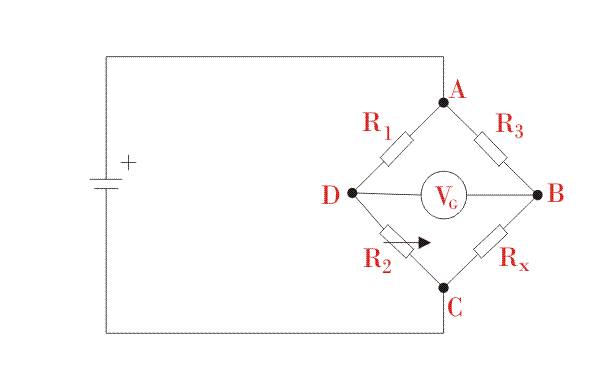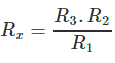## Maxwell’s Bridge

The working principle of the Maxwell’s inductance bridge is same as the Wheatstone bridge. Only little modifications have been done in Wheatstone bridge. In this bridge, the four branches consist of unknown inductance (L1), a variable capacitor (C4), four resistors and detector instead of galvanometer as shown in the figure. It is used to measure the value of inductance by comparing the unknown value with the standard variable capacitance.

The basic principle of the bridge is to compensate the positive angle phase of the unknown impedance with the negative phase of a capacitance by putting it in opposite branch. By doing so, the potential difference across the detector will become zero and no current will flow through it. The capacitor C4 and resistor R4 are connected in parallel and the value of both are adjusted so that bridge get balanced.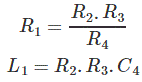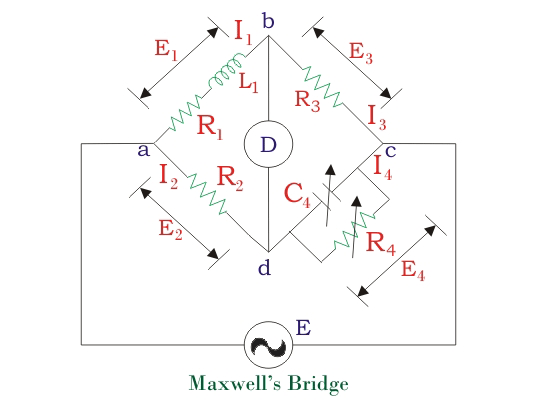## Kelvin Bridge

Kelvin Bridge is another modification of the Wheatstone bridge which is used to measure low resistance in the range of 1mΩ to 1kΩ with great accuracy. For precise measurement of low resistance, high voltage supply and a sensitive galvanometer are required in Kelvin Bridge. While measuring low resistance, the resistance of connecting wires plays an important role. Wheatstone bridge is used which has two additional resistors as shown in the figure. The resistors R1 and R2 are connected to the second set of ratio-arm and constructed four terminal resistors. Here R is unknown and S is the standard resistor. A galvanometer is placed between c and d so that resistance of connecting wire r can be neglected and does not affect the measurement value. Under the balance condition, galvanometer shows zero and no current flows through the circuit. The equation at balance condition is: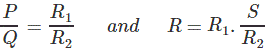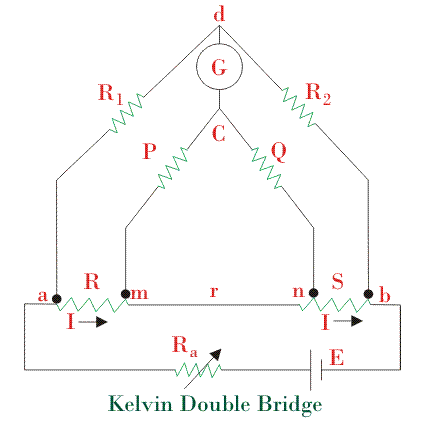## Hay’s Bridge Circuit

Hay’s bridge is another variation of Maxwell’s bridge circuit. In Maxwell’s circuit resistance is kept parallel to the capacitor where as, in Hay’s circuit, the resistor is connected in series with the standard capacitor as shown in the figure. It is very useful if the phase angle of inductive impedance is very large, which could be overcome by taking a low resistance in series.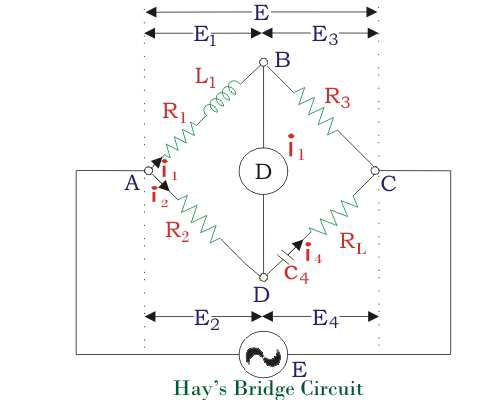## Anderson’s Bridge

Anderson Bridge is modified version of Maxwell’s inductor capacitance bridge. It is mainly used for measuring self-inductance in a coil by using standard capacitor and resistors. The main advantage of this bridge is that it does not require the frequent balancing of the bridge. To balance the bridge by steady current, variable resistance r is adjusted and AC source is replaced by battery and headphone by moving coil galvanometer. Once the bridge is balanced the potential at the terminal D is similar to the potential at E. the flow of current in respective branches are denoted by I1, I2, and I3 as shown in the figure.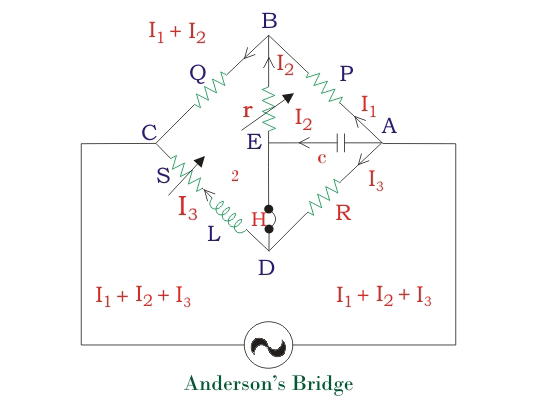## Diode Bridge Circuit

It is a bridge circuit having an arrangement of four diodes that gives the same output polarity for every input polarity. Diode bridge circuit which also called bridge rectifier is used where ever there is a need to change alternating current into direct current. It is also used to detect the amplitude of radio signals. When the positive terminal of the input is connected to the upper left and negative to the lower right, the current flows from upper supply terminal to the output flowed by red path and returns back to the lower supply terminal through the blue path as shown in the figure.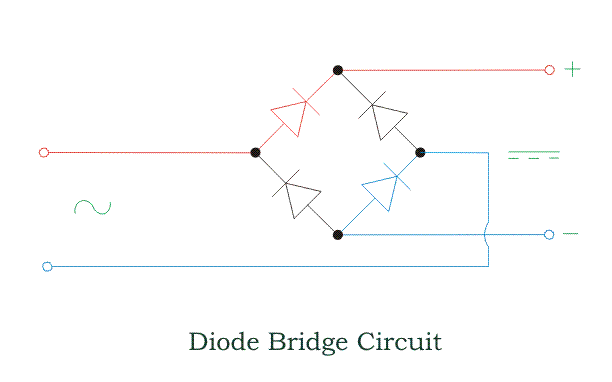Want To Learn Faster? 🎓
Get electrical articles delivered to your inbox every week.
No credit card required—it’s 100% free.